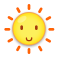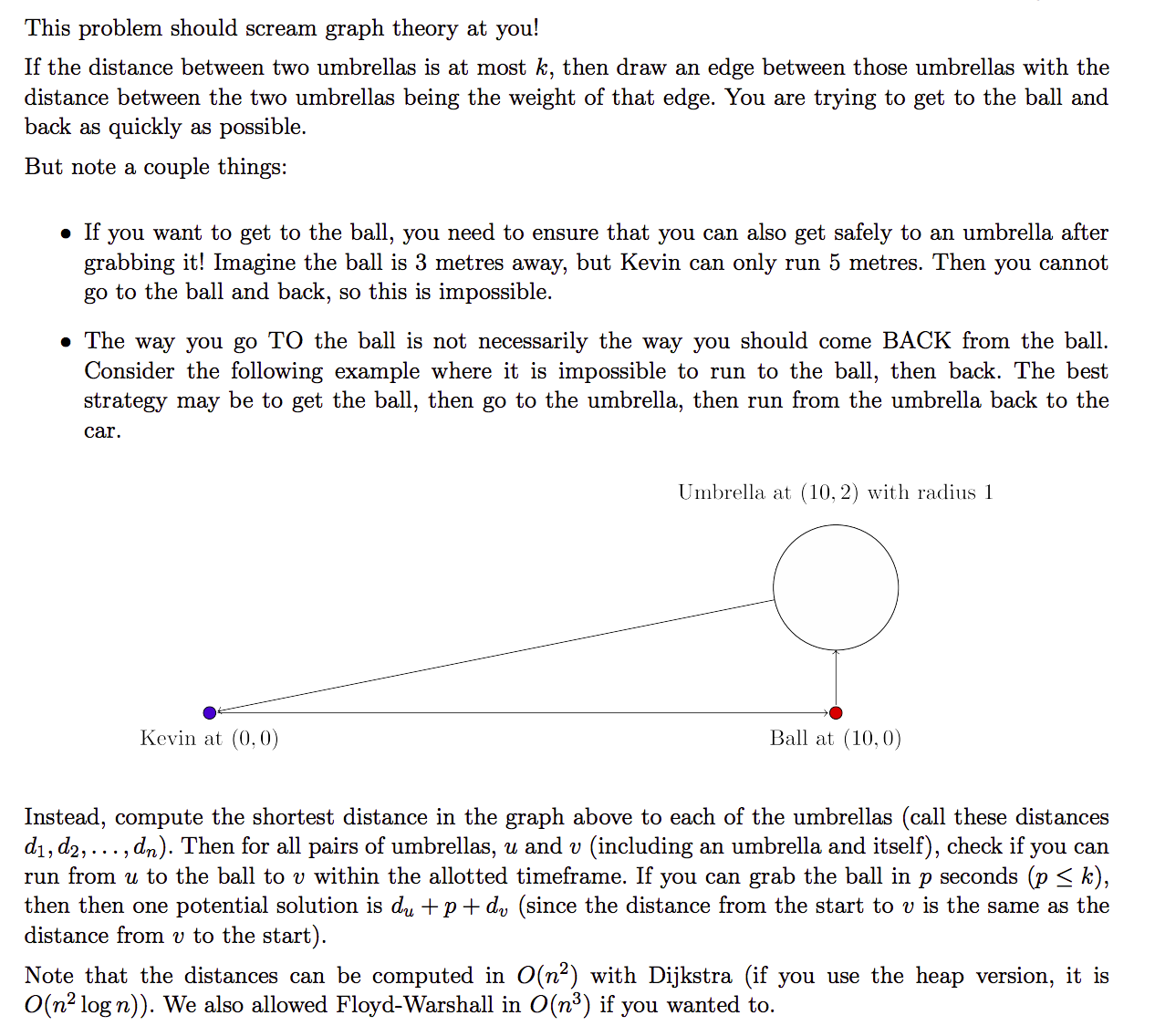# CSU2110 Keeping Cool（最短路）

## Keeping Cool

### Description

Kevin has just gotten back to his car after a morning at the beach and is about to drive away when he realises that he has left his ball somewhere. Thankfully, he remembers exactly where it is! Unfortunately for Kevin, it is extremely hot outside and any sand that is exposed to direct sunlight is very hot. Kevin’s pain tolerance allows him to only run for at most k seconds in the hot sand at one time. Kevin runs at exactly 1 metre per second on hot sand. Scattered around the beach are umbrellas. Each umbrella is a perfect circle and keeps the sand underneath it cool. Each time Kevin reaches an umbrella, he will wait there until his feet cool down enough to run for another k seconds on the hot sand. Note that Kevin will not run more than k seconds in the hot sand at one time, so if two umbrellas are more than k metres apart, Kevin will not run between them. Determine the minimum amount of time that Kevin must be in the sun in order to retrieve his ball and return back to the car.### Input

The first line of input contains four integers n (0 ≤ n ≤ 100), which is the number of umbrellas, k (1 ≤ k ≤ 100), which is the number of metres that Kevin can run on the hot sand, x (−100 ≤ x ≤ 100) and y (−100 ≤ y ≤ 100), which are the coordinates of the beach ball. Kevin starts at his car at (0; 0). You may treat Kevin and the ball as single points. The next n lines describe the umbrellas. Each of these lines contains three integers x (−100 ≤ x ≤ 100), y (−100 ≤ y ≤ 100) and r (1 ≤ r ≤ 100). The umbrella is a circle centred at (x; y) with radius r. There may be multiple items (ball, umbrella(s) or Kevin) at a single location. All measurements are in metres.

### Output

Display the minimum amount of time (in seconds) that Kevin must be in the sun. If it is impossible for Kevin to get to the ball and return back to the car, display -1 instead. Your answer should have an absolute or relative error of less than 10−6.

### Sample Input

0 1 0 0

0 20 1 2

0 10 20 20

2 2 7 4
6 2 2
2 2 1

1 2 3 3
0 3 2

### Sample Output

0.0000000000

4.4721359550

-1

6.1289902045

4.0000000000

### 链接

https://cn.vjudge.net/problem/CSU-2110

### 题意

• 如果两把伞之间的距离小于等于k，那么它们可以互相到达
• 在遮阳伞的范围之内休息不算在太阳下走的时间

### 题解

• 小K在捡到球后能否成功返回，0 5 3 0应该输出-1而不是6
• 返回的路径与出发的路径并不一定相同1 6 5 0 5 2 1应该输出10.38...而不是10.77...

### 代码

StatusAccepted
Time4ms
Memory2132kB
Length3353
#include <iostream>
#include <cstdio>
#include <algorithm>
#include <cstring>
#include <cmath>
using namespace std;

const double eps = 1e-15;
const int inf = 0x3f3f3f3f;
const int maxn = 110;
double G[maxn][maxn];
double dis[maxn];
bool vis[maxn];
struct node
{
int x, y, r;
}a[maxn];
int s, t;

double dist(double x1, double y1, double x2, double y2)
{
return sqrt((x2 - x1) * (x2 - x1) + (y2 - y1) * (y2 - y1));
}

void dijkstra(int n)
{
for (int i = 0; i <= n; ++i)
{
dis[i] = inf * 1.0;
vis[i] = 0;
}
dis[s] = 0.0;
for (int i = 0; i <= n; ++i)
{
double Min = inf * 1.0;
int k = -1;
for (int j = 0; j <= n; ++j)
{
if (dis[j] < Min && !vis[j])
{
Min = dis[j];
k = j;
}
}
if (k == -1)
break;
vis[k] = true;
for (int j = 0; j <= n; ++j)
{
if (G[k][j] + Min < dis[j] && !vis[j])
dis[j] = Min + G[k][j];
}
}
}

int main()
{
int n, k, x, y;
scanf("%d%d%d%d", &n, &k, &x, &y);
s = 0, t = n + 1;
for (int i = 0; i <= n + 1; ++i) // 初始化
for (int j = 0; j <= n + 1; ++j)
G[i][j] = ((i == j) ? 0.0 : inf * 1.0);
double d0 = dist(0.0, 0.0, x * 1.0, y * 1.0);
if (d0 * 2 <= k * 1.0) // 起点与球建边
G[n+1] = G[n+1] = d0;
for (int i = 1; i <= n; ++i)
scanf("%d%d%d", &a[i].x, &a[i].y, &a[i].r);
for (int i = 1; i <= n; ++i) // 伞与起点和终点建边
{
double d1 = dist(0.0, 0.0, a[i].x * 1.0, a[i].y * 1.0) - a[i].r * 1.0;
double d2 = dist(x * 1.0, y * 1.0, a[i].x * 1.0, a[i].y * 1.0) - a[i].r * 1.0;
if (d1 <= 0.0)
G[i] = G[i] = 0.0;
else if (d1 <= k * 1.0)
G[i] = G[i] = d1;
if (d2 <= 0.0)
G[n+1][i] = G[i][n+1] = 0.0;
else if (d2 <= k * 1.0)
G[n+1][i] = G[i][n+1] = d2;
}
for (int i = 1; i <= n; ++i) // 伞间建边
for (int j = 1; j <= n; ++j)
if (j != i)
{
double d = dist(a[i].x, a[i].y, a[j].x, a[j].y) - (a[i].r + a[j].r) * 1.0;
if (d <= 0.0)
G[i][j] = G[j][i] = 0.0;
else if (d <= k * 1.0)
G[i][j] = G[j][i] = d;
}
dijkstra(n);
double ans = G[n+1] * 2.0;
for (int i = 1; i <= n; ++i)
{
for (int j = 1; j <= n; ++j) // 起点->伞->球->伞->起点
{
if (G[i][n+1] + G[j][n+1] <= k * 1.0)
ans = min(ans, dis[i] + dis[j] + G[i][n+1] + G[j][n+1]);
}
if (d0 + G[n+1][i] <= k * 1.0) // 起点->球->伞->起点
ans = min(ans, d0 + G[n+1][i] + dis[i]);
}
if (ans - (inf * 1.0) > eps)
printf("-1\n");
else
printf("%.10f\n", ans);
return 0;
}The end.
2018-05-23 星期三

1.太傅

重新写一遍花了我十几分钟 @(呵呵)